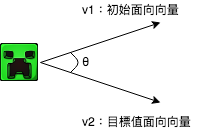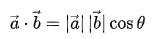#2019 iT 邦幫忙鐵人賽DAY 13
2
Modern Web

## 用 Three.js 來當個創世神 (12)：專案實作#7 - Tween.js 動畫實作Photo by Denise Jans on Unsplash

## 今日目標## Tween.js 簡介

Tween.js 是一個輕量的 Javascript 函式庫，可以輕易地做到補間動畫（tween），只要給它起始值、結束值、過程需花費多少時間，其他的中間值會自動被計算出來。

``````var coords = { x: 0, y: 0 }; // 起點為 (0, 0)
var tween = new TWEEN.Tween(coords) // 建立新的 Tween 物件來改變 coords
.to({ x: 300, y: 200 }, 1000) // 在 1000ms 內移動至（300, 200）
.onUpdate(function() { // 在 coords 被改變時會執行的 callback function
...
})
.start(); // 立即啟動 tween 動畫
``````

Codepen demo

`Tween.js` 有興趣的讀者可以參考以下資源：

## 專案實作

### 引入 Tween.js

``````https://cdnjs.cloudflare.com/ajax/libs/tween.js/17.2.0/Tween.min.js
``````

### 起始值設定

``````let offset = { x: 0, z: 0, rotateY: 0 }
let target = { x: 20, z: 20, rotateY: 0.7853981633974484 } // 目標值
``````

### 在原點與相機間往返運動

``````// 朝相機移動
tween = new TWEEN.Tween(offset)
.to(target, 3000)
.onUpdate(onUpdate)
.onComplete(() => {
tweenBack.start()
})

// 回原點
tweenBack = new TWEEN.Tween(offset)
.to({ x: 0, z: 0, rotateY: 0 }, 3000)
.onUpdate(onUpdate)
.onComplete(() => {
handleNewTarget() // 計算新的目標值
tween.start()
})
``````

`.onComplete()` 中是當動畫完成後要做的處理，在 `tween` 中的比較簡單，只要觸發 `tweenBack` 即可；而在 `tweenBack` 中則要去準備下一階段要從原點出發的目標值再觸發 `tween` 一直循環下去。

### 準備新的目標值

``````// 計算新的目標值
const handleNewTarget = () => {
// 限制苦力怕走路邊界
if (camera.position.x > 30) target.x = 20
else if (camera.position.x < -30) target.x = -20
else target.x = camera.position.x

if (camera.position.z > 30) target.z = 20
else if (camera.position.z < -30) target.z = -20
else target.z = camera.position.z

const v1 = new THREE.Vector2(0, 1) // 原點面向方向
const v2 = new THREE.Vector2(target.x, target.z) // 苦力怕面向新相機方向

// 內積除以純量得兩向量 cos 值
let cosValue = v1.dot(v2) / (v1.length() * v2.length())

// 防呆，cos 值區間為（-1, 1）
if (cosValue > 1) cosValue = 1
else if (cosValue < -1) cosValue = -1

// cos 值求轉身角度
target.rotateY = Math.acos(cosValue)
}
````````````// 內積除以純量得兩向量 cosθ
let cosValue = v1.dot(v2) / (v1.length() * v2.length())

// 防呆，cosθ 區間為（-1, 1）
if (cosValue > 1) cosValue = 1
else if (cosValue < -1) cosValue = -1

// cosθ 求轉身角度
target.rotateY = Math.acos(cosValue)
``````

### 補間動畫

``````// 苦力怕走動及轉身補間動畫
const onUpdate = () => {
// 移動
creeperObj.feet.position.x = offset.x
creeperObj.feet.position.z = offset.z
creeperObj.body.position.x = offset.x
creeperObj.body.position.z = offset.z

// 轉身
if (target.x > 0) {
creeperObj.feet.rotation.y = offset.rotateY
creeperObj.body.rotation.y = offset.rotateY
} else {
creeperObj.feet.rotation.y = -offset.rotateY
creeperObj.body.rotation.y = -offset.rotateY
}
}
``````

### 其他設定

``````function createCreeper() {
creeperObj = new Creeper()
tweenHandler() // 追蹤相機動畫
}
``````

``````gui.add(datGUIControls, 'startTracking').onChange(function(e) {
startTracking = e
if (invert > 0) { // invert = 1, tween
if (startTracking) {
tween.start()
} else {
tween.stop()
}
} else { // invert = -1, tweenBack
if (startTracking) {
tweenBack.start()
} else {
tweenBack.stop()
}
}
})
``````

``````function render() {
...
TWEEN.update() // update
...
requestAnimationFrame(render)
renderer.render(scene, camera)
}
``````

## 今日小結

### 1 則留言

1

Dez大大有用過three.js/editorNova Skin嗎？

CK Chuang iT邦新手 4 級 ‧ 2018-10-28 14:17:37 檢舉